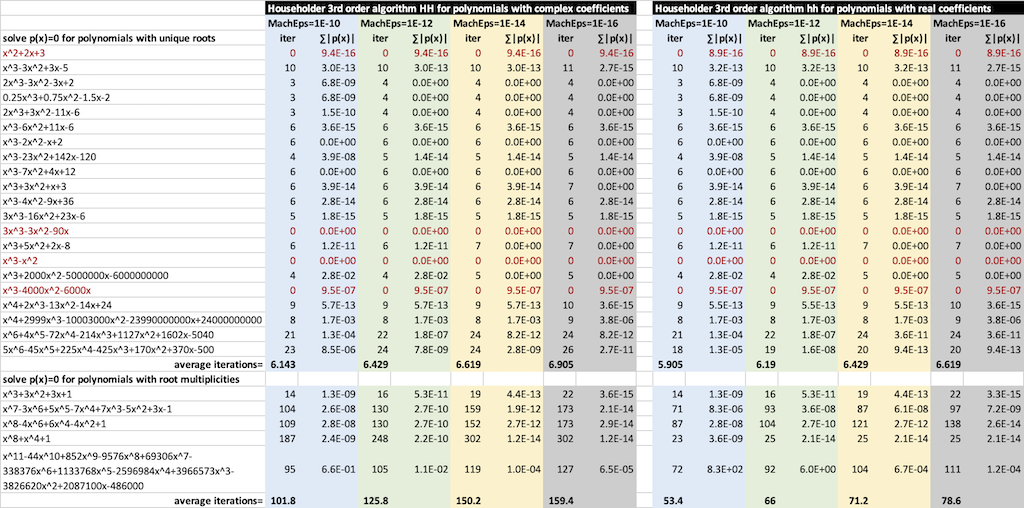(PC-12xx~14xx) Householder 3rd order method
03-10-2022, 02:01 AM (This post was last modified: 03-10-2022 02:03 AM by robve.)
Post: #2
 robveSenior Member Posts: 395 Joined: Sep 2020
RE: (PC-12xx~14xx) Householder 3rd order method
Shown below is a comparison of Householder 3rd order method for polynomials with complex coefficients (algorithm "HH") and the method for polynomials with real coefficients (algorithm "hh"). For each of the four given MachEps values, the total number of iterations iter to converge the roots is shown, together with the sum of the residual absolute errors. Note that the last root or last two roots are computed directly, hence the polynomials shown in red font require no iterations. Note that the residual errors are expected to typically larger for polynomials of higher order with large coefficients, since a small change in the root z may cause a large change in p(z).For the algorithms, I came up with the following idea to use a simple starting point choice for the complex root $$z$$ of the (deflated) polynomial of order $$m$$:
$$z.re = \frac{a_m}{\sqrt{2}\,a_{m-1}}$$ if $$a_{m-1} \neq 0$$ otherwise $$z.re = \frac{1}{\sqrt{2}}$$
$$z.im = \frac{z.re}{m}$$.
Note: $$a_m$$ is the last coefficient, i.e. $$p(z)=...+a_{m-2}z^2+a_{m-1}z+a_m$$
Finding the roots in increasing order of magnitude is combined with forward deflation to improve numerical stability. This choice of starting point appears to perform very well, especially for polynomials with root multiplicities, but I have no analytic proof that explains why this is the case.

Choosing a root modulus error tolerance is key to converge to an acceptable accuracy without "overdoing" the iterations:
$$\epsilon = 6\,m\,|a_m|\,\mbox{MACHEPS}$$.
The stopping criterion is simply:
$$|p(z)|<\epsilon$$ and $$|h|\le(|r|+1)\,\mbox{MACHEPS}$$.
This is more efficient to compute with the squared magnitudes (allowing a modest numerical difference in the formula):
$$|p(z)|^2<\epsilon^2$$ and $$|h|^2\le(|r|^2+1)\,\mbox{MACHEPS}^2$$
We pick $$|r|^2+1$$ to bound the error to ~MACHEPS if $$|r|<1$$.

The algorithm checks if the root $$z$$ is real-valued instead of complex if the imaginary part is negligible or if $$|p(z.re)|^2\le|p(z)|^2$$.

The "hh" version is optimized for polynomials with real coefficients. Therefore, two roots (the root and its conjugate) are found at a time if the root has a nonzero imaginary part.

- Rob

"I count on old friends" -- HP 71B,Prime|Ti VOY200,Nspire CXII CAS|Casio fx-CG50...|Sharp PC-G850,E500,2500,1500,14xx,13xx,12xx...
 « Next Oldest | Next Newest »

 Messages In This Thread (PC-12xx~14xx) Householder 3rd order method - robve - 03-10-2022, 01:24 AM RE: (PC-12xx~14xx) Householder 3rd order method - robve - 03-10-2022 02:01 AM

User(s) browsing this thread: 1 Guest(s)